Question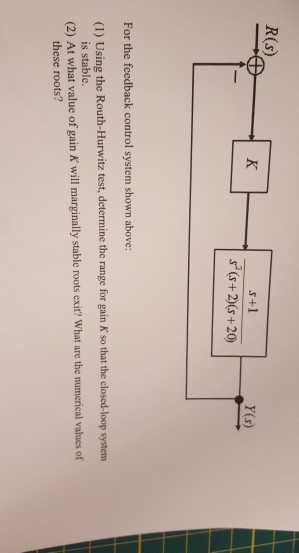question from analysis and design of control systems subject help please!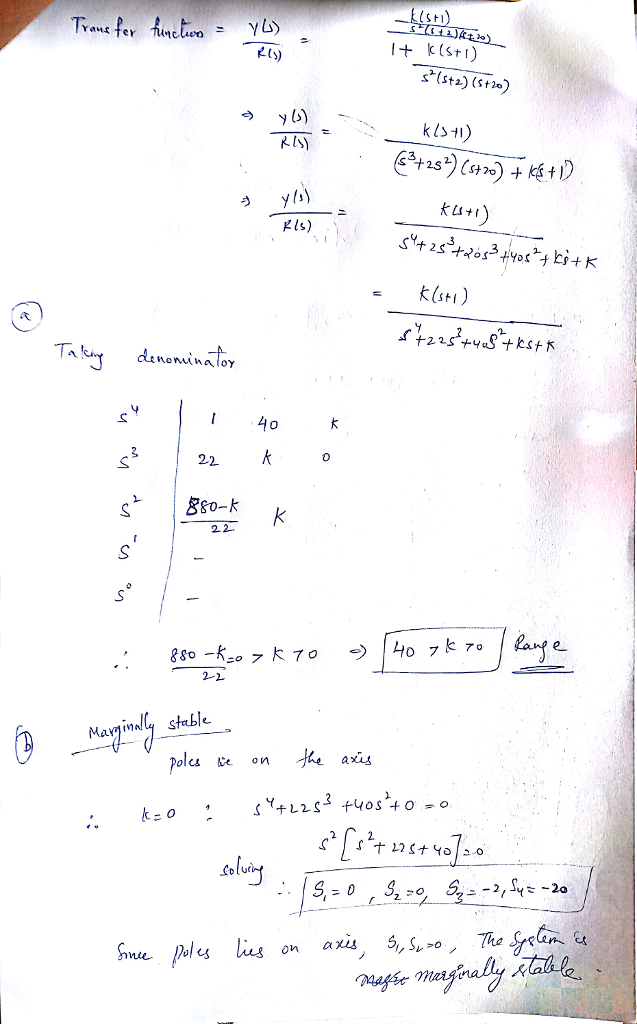#### Earn Coins

Coins can be redeemed for fabulous gifts.

Similar Homework Help Questions
• ### Question 2 System Stability in the s-Domain and in the Frequency Domain: Bode Plots, Root Locus...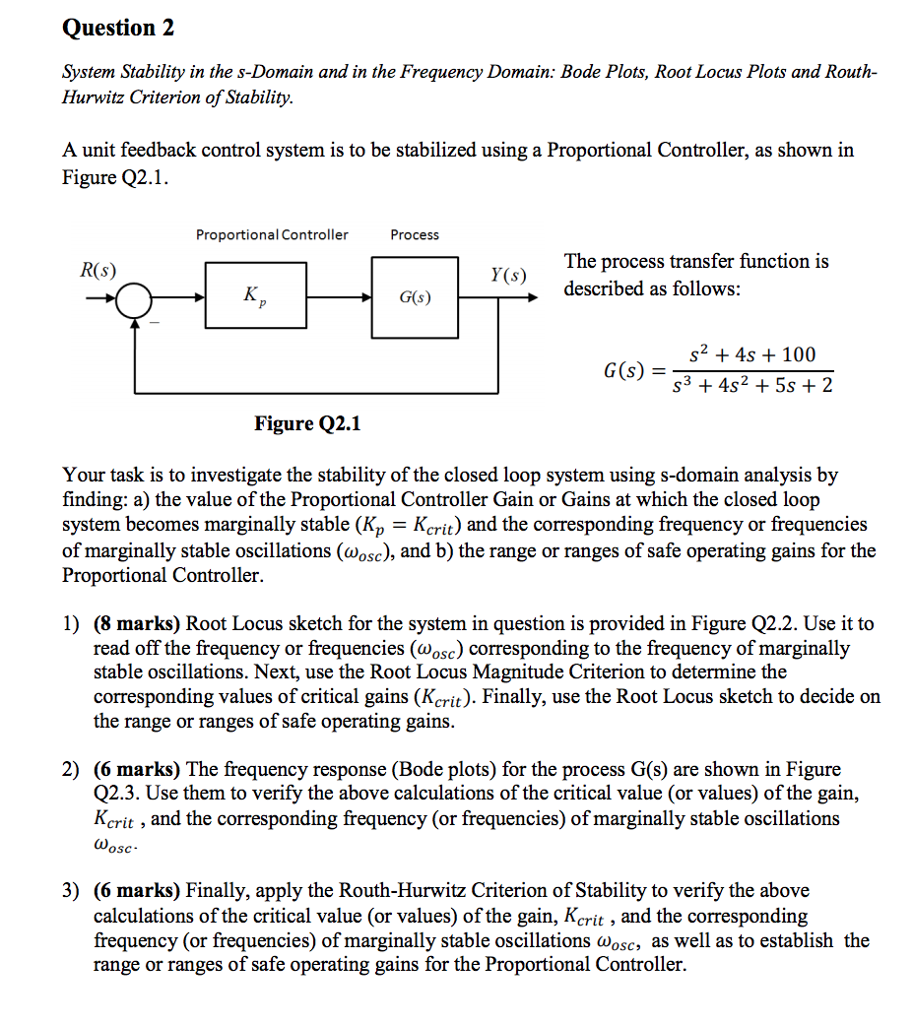Question 2 System Stability in the s-Domain and in the Frequency Domain: Bode Plots, Root Locus Plots and Routh- Hurwitz Criterion ofStability A unit feedback control system is to be stabilized using a Proportional Controller, as shown in Figure Q2.1. Proportional Controller Process The process transfer function is described as follows: Y(s) G(s) s2 +4s 100 s3 +4s2 5s 2 Figure Q2.1 Your task is to investigate the stability of the closed loop system using s-domain analysis by finding: a)...

• ### 1. Consider the usual unity-feedback closed-loop control system with a proportional-gain controll...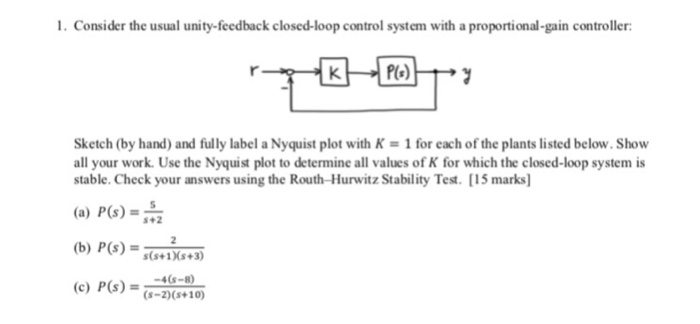1. Consider the usual unity-feedback closed-loop control system with a proportional-gain controller Sketch (by hand) and fully label a Nyquist plot with K-1 for each of the plants listed below.Show all your work. Use the Nyquist plot to determine all values of K for which the closed-loop system is stable. Check your answers using the Routh-Hurwitz Stability Test. [15 marks] (a) P(s)-2 (b) P(s)-1s3 (c) P(s) -4-8 s+2 (s-2) (s+10) 1. Consider the usual unity-feedback closed-loop control system with a...

• ### Question 6 The open-loop transfer function G(s) of a control system is given as G(8)- s(s+2)(s +5...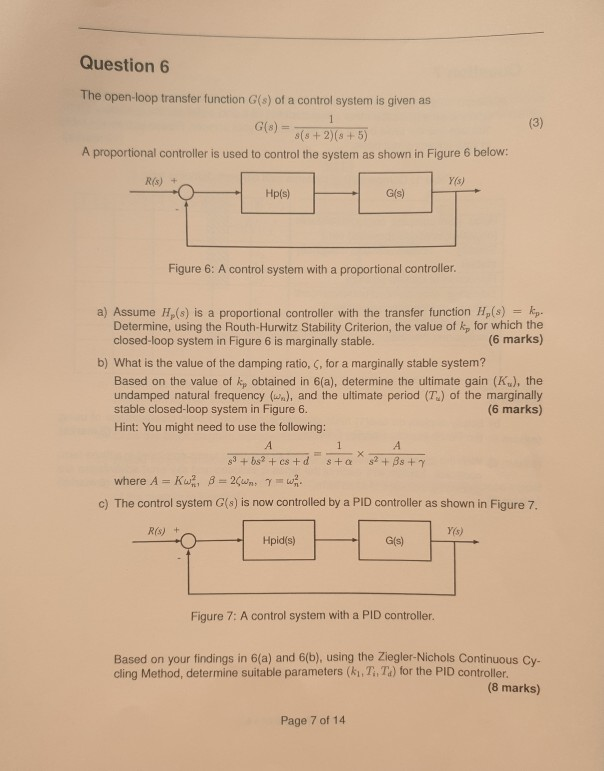Question 6 The open-loop transfer function G(s) of a control system is given as G(8)- s(s+2)(s +5) A proportional controller is used to control the system as shown in Figure 6 below: Y(s) R(s) + G(s) Figure 6: A control system with a proportional controller a) Assume Hp(s) is a proportional controller with the transfer function H,(s) kp. Determine, using the Routh-Hurwitz Stability Criterion, the value of kp for which the closed-loop system in Figure 6 is marginally stable. (6...

• ### Let G,()+3s+5) , K-1 and Ge 1 I Determine the loop transfer function L(s)-KG.G. Use 'margin' command in matlab to generate the Bode Plot for L(s). (a) What are its gain and phase margins (the...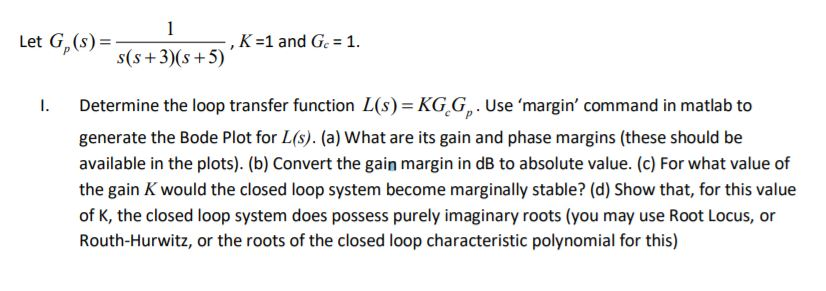Let G,()+3s+5) , K-1 and Ge 1 I Determine the loop transfer function L(s)-KG.G. Use 'margin' command in matlab to generate the Bode Plot for L(s). (a) What are its gain and phase margins (these should be available in the plots). (b) Convert the gain margin in dB to absolute value. (c) For what value of the gain K would the closed loop system become marginally stable? (d) Show that, for this value of K, the closed loop system does...

• ### 1. Consider the usual unity-feedback closed-loop control system with a proportional-gain controller: 19 r - PGS-Try...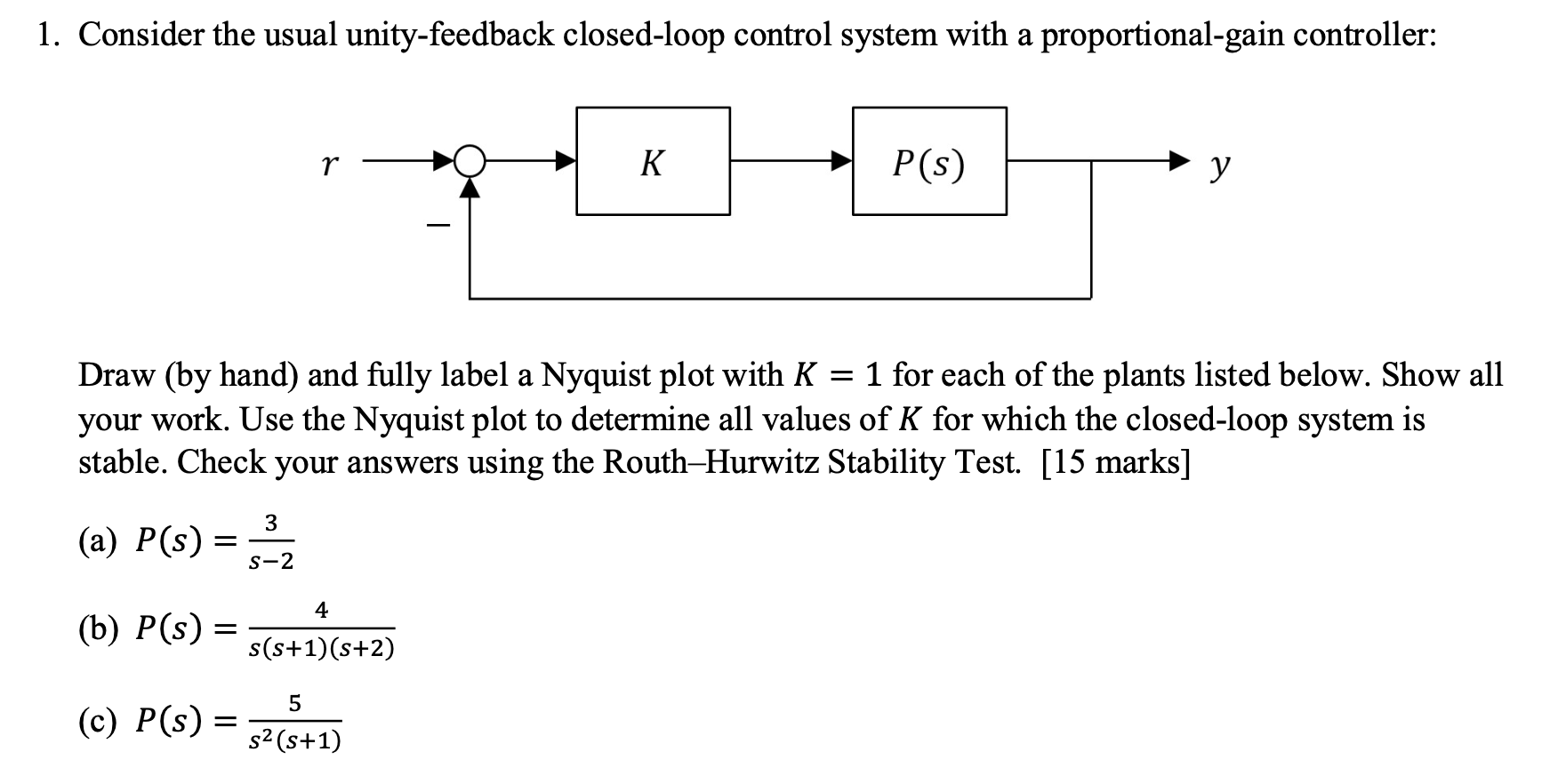1. Consider the usual unity-feedback closed-loop control system with a proportional-gain controller: 19 r - PGS-Try P(s) Draw (by hand) and fully label a Nyquist plot with K = 1 for each of the plants listed below. Show all your work. Use the Nyquist plot to determine all values of K for which the closed-loop system is stable. Check your answers using the Routh-Hurwitz Stability Test. [15 marks] (a) P(s) = (b) P(s) = s(s+13 (6+2) (©) P(s) = 32(6+1)

• ### Feedback Control of Dynamic System Please Let me know how to solve this problem (5) For the following unity-feedback control system, Y(s) R(s)E D(s) (s+ 2) we want to design a controller D(s) D(s)+a)...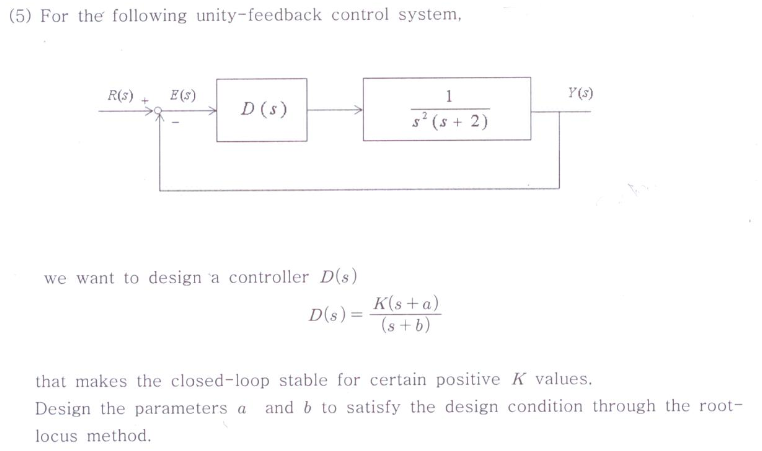Feedback Control of Dynamic System Please Let me know how to solve this problem (5) For the following unity-feedback control system, Y(s) R(s)E D(s) (s+ 2) we want to design a controller D(s) D(s)+a) that makes the closed-loop stable for certain positive K values. Design the parameters a and b to satisfy the design condition through the root- locus method (5) For the following unity-feedback control system, Y(s) R(s)E D(s) (s+ 2) we want to design a controller D(s) D(s)+a)...

• ### Consider the feedback sy PID COntroller Plant R(S) Y(s) the closed-loop transfer function T(s) = Y...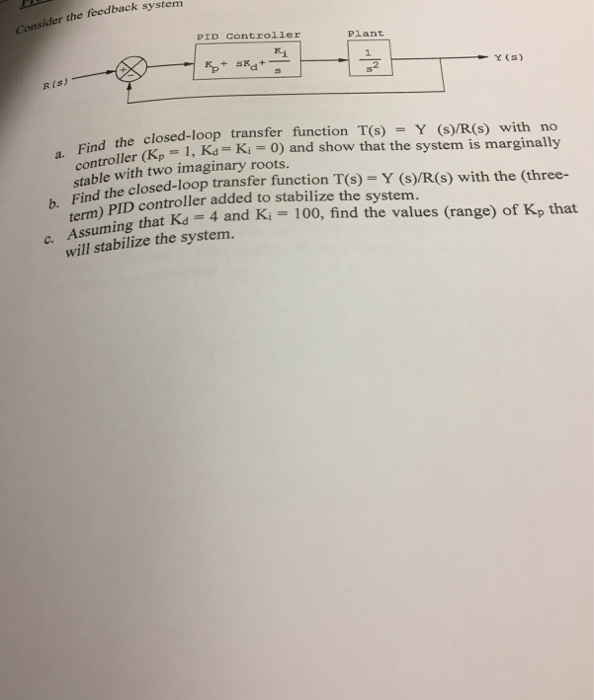Consider the feedback sy PID COntroller Plant R(S) Y(s) the closed-loop transfer function T(s) = Y controller (Kp Find er p 1, Ks K ) and show that the system is marginally stable with two imaginary roots. (s)/R(s) with no sabl thosed-loop transfer function T(s) Y (S/R(s) with the (three- term) PID controller added to stabilize the system. suming that Kd 4 and K, -100, find the values (range) of Kp that will stabilize the system.

• ### 2. From the following Closed-Loop Transfer Function, find the range of Gain K that will cause...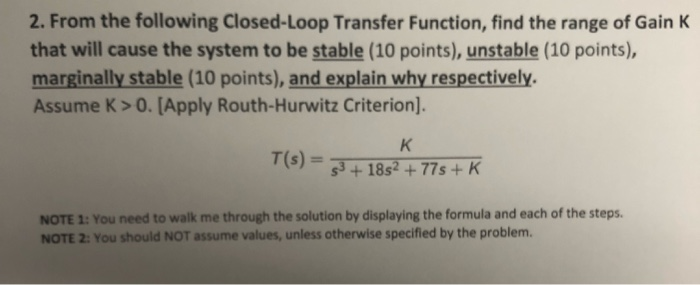2. From the following Closed-Loop Transfer Function, find the range of Gain K that will cause the system to be stable (10 points), unstable (10 points), marginally stable (10 points), and explain why respectively Assume K>O.[Apply Routh-Hurwitz Criterion NOTE 1: You need to walk me through the solution by displaying the formula and each of the steps. NOTE 2: You should NOT assume values, unless otherwise specified by the problem.

• ### 3. For the feedback control system shown in Figure Q3 below, the forward-path transfer function given...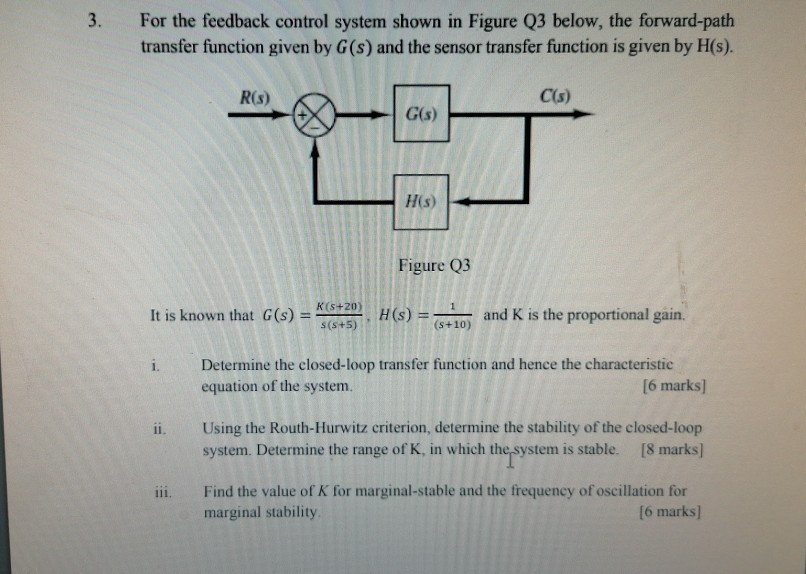3. For the feedback control system shown in Figure Q3 below, the forward-path transfer function given by G(s) and the sensor transfer function is given by H(s). R(s) C(s) G(s) H(s) Figure Q3 It is known that G(s) -- K(+20) S(+5) H(s) = and K is the proportional gain. (S+10) i. Determine the closed-loop transfer function and hence the characteristic equation of the system. [6 marks] ii. Using the Routh-Hurwitz criterion, determine the stability of the closed-loop system. Determine the...

• ### Q2 (a) List down THREE (3) important requirements to design a control system. (3 marks) State...Q2 (a) List down THREE (3) important requirements to design a control system. (3 marks) State the possible consequence when a physical system becomes unstable. (2 marks) (6) (c) Consider the following characteristic Equation shown below: P(s) = 55 +683 + 582 +8s + 20 (1) Construct Routh table for the characteristic Equation. (6 marks) (ii) Using the Routh – Hurwitz criterion, determine the stability of the system. (2 marks) (ii) Determine the numbers of roots on the right half-plane,...